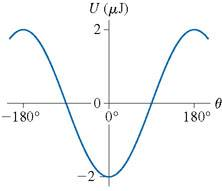# Energy of a Dipole in a Constant E Field

## Homework Statement

The graph shows the potential energy of an electric dipole which is in a constant electric field; only the electric force is acting on the dipole. Consider a dipole that oscillates between plus and minus 65 degrees.a) What is the dipole's mechanical energy?
b) What is the dipole's kinetic energy when it is aligned with the electric field?

## Homework Equations

U = -pEcos$$\theta$$

## The Attempt at a Solution

I know that when the graph is at the trough it's -pE and at it's peak it's +pE. But I don't know how to factor in the 65 degrees. Please help!

## Answers and Replies

rl.bhat
Homework Helper
At 180 degrees U = 2 μJ. Substitute in the relevant equation and find pE.
At θ = 0, all the potential energy in converted to K.E. Similarly you can find P.E. at 65 degrees by substituting the value of pE and θ in the equation.

At 180 degrees U = 2 μJ. Substitute in the relevant equation and find pE.
At θ = 0, all the potential energy in converted to K.E. Similarly you can find P.E. at 65 degrees by substituting the value of pE and θ in the equation.

Thank you so much! That really cleared it up for me!